Latest Banking jobs   »   Quantitative Aptitude Quiz For SBI Clerk...

# Quantitative Aptitude Quiz For SBI Clerk Prelims 2021- 16th May

Q1. A train can cross a platform of 100 m length completely in 12 seconds while covers a platform of double of its length in 21 seconds. Find the speed of the train? (in m/s)
(a) 12
(b)15
(c) 18
(d) 20
(e) 24

Q2. The circumference of two circles is 132 m and 176 m respectively. What is difference between the area of larger circle and smaller circle ? (in m²)
(a) 1052
(b) 1128
(c) 1258
(d) 1078
(e) 1528

Q3. Diameter of a cylindrical jar is increased by 25%. By what percent must the height be decreased so that there is no change in its volume?
(a) 18%
(b) 25%
(c) 32%
(d) 36%
(e) None of these

Q4. If speed of boat in upstream is double than the speed of current and speed of boat in still water is 27 km/hr. Then find the time taken by boat to travel 54 km downstream? (in hour)
(a) 1.5
(b) 1.8
(c) 2.5
(d) 1.2
(e) 2

Q5. The letters of the word PROMISE are to be arranged so that three vowels should not come together. Find the number of arrangements.
(a) 4470
(b) 4320
(c) 3792
(d) 4200
(e) 4450

Q6. Train A is 180 metre long, while another train B is 240 metre long. Train A has a speed of 30 kmph and train B’s speed is 40 kmph, if the trains move in opposite directions then in what time will Train A pass Train B completely?
(a) 21 seconds
(b) 21.6 seconds
(c) 26.1 seconds
(d) 26 seconds
(e) 16 seconds

Q7. A train covers certain distance between two places at a uniform speed. If the train moved 10 kmph faster, it would take 2 hours less, and if the train were slower by 10 kmph, it would take 3 hours more than the scheduled time. Find the distance covered by the train.
(a) 300 km
(b) 600 km
(c) 800 km
(d) 1200 km
(e) None of these

Q8. How many five-letters containing 2 vowels and 3 consonants can be formed using the letters of the word EQUALITY so that 2 vowels occur together?
(a) 1260
(b) 1000
(c) 1150
(d) 1152
(e) None of these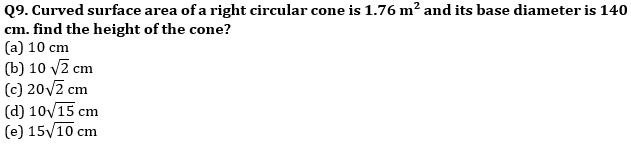Q10. A man can row 15 kmph in still water and it takes him 75 minutes to row to a place and back if the speed of current is 3 Kmph, then how far is the place?
(a) 9 km
(b) 6 km
(c) 12 km
(d) 15 km
(e) 13.5 km

Q11. There are 5 multiple choice questions in an examination. How many sequences of answers are possible, if the first three questions have 4 choices each and the next two have 6 choices each?
(a) 2804
(b) 3456
(c) 7776
(d) 2304
(e) 1024

Q12. What will be the cost of fencing the rectangular field whose area is 486 sq. m. if the cost of fencing is Rs 11 per meter and length of the field is 50% more than the breadth of the field.
(a) Rs 1100
(b) Rs 990
(c) Rs 880
(d) Rs 770
(e) Rs. 660

Q13. Ratio of upstream speed to downstream speed is 1 : 11. If speed of boat is 30 km/hr. Find the distance covered in upstream in 5 hours ? (in km)
(a) 66
(b) 55
(c) 25
(d) 30
(e) 40

Q14. What will be the difference between simple interest and compound interest at 10% p.a. on the sum of Rs 10,000 after 4 years?
(a) 628
(b) 541
(c) 640
(d) 540
(e) 641

Q15. Mohan has two sons named Ram and Karan. The ratio of age of Mohan and Ram is 5 : 2 and that of Ram and Karan is 8 : 5. Also, Ram is 6 years elder than Karan. Find the ratio of their ages after 10 years.
(a) 23 : 10 : 4
(b) 25 : 16 : 9
(c) 25 : 13 : 10
(d) 50 : 26 : 19
(e) 25 : 16 : 10

Q16. Pipe A and Pipe B can fill a cistern together in 18 minutes. Pipe B is 50% more efficient than pipe A. Find the capacity of cistern, if it is given that pipe ‘A’ fills the cistern at speed of 6 litre/minute.
(a) 150 l
(b) 225 l
(c) 240 l
(d) 180 l
(e) 270 l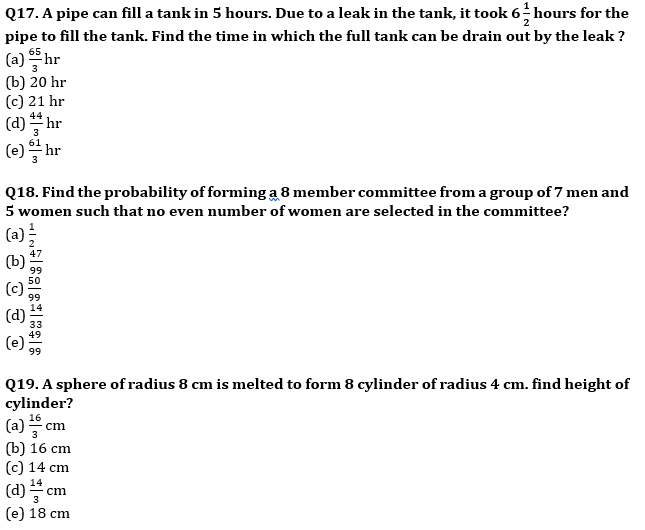Q20. A sum of money of Rs. 8000 is invested at rate of 40% p.a. for 1 year. Find CI if rate is compounded quarterly.
(a) Rs. 3600
(b) Rs. 3712.8
(c) Rs. 3625.18
(d) Rs. 3576.8
(e) Rs. 4200

Practice More Questions of Quantitative Aptitude for Competitive Exams:

###### SBI Clerk Study Plan 2021

Solutions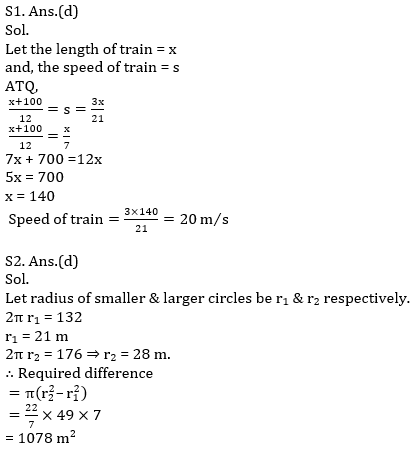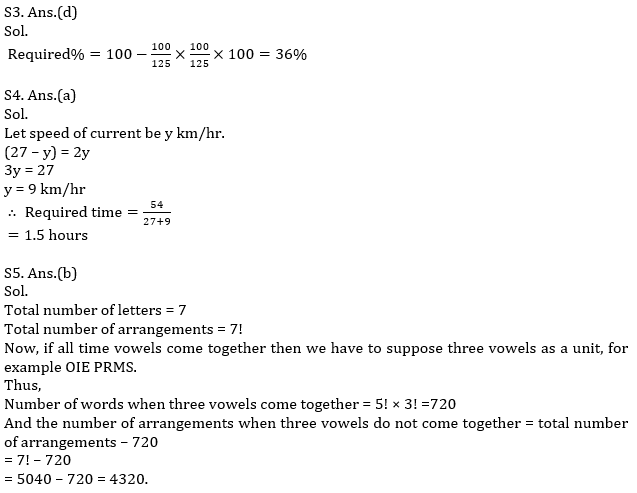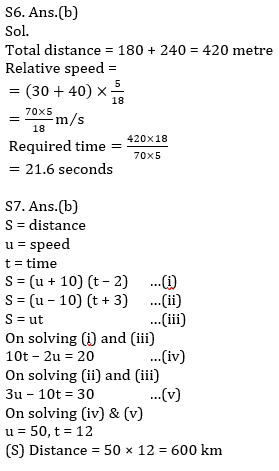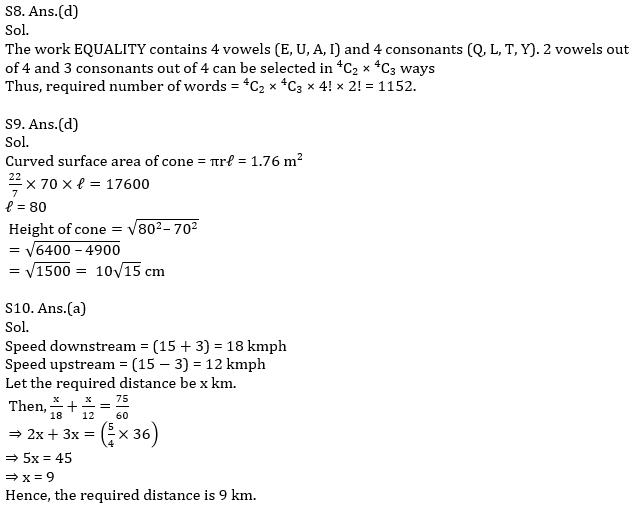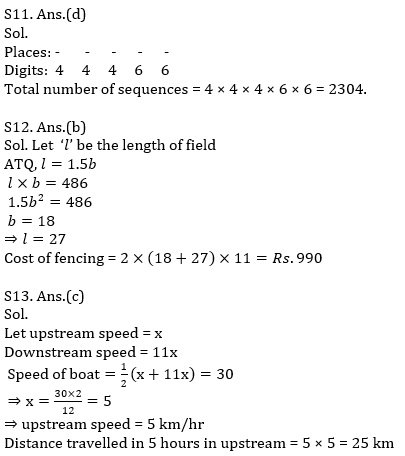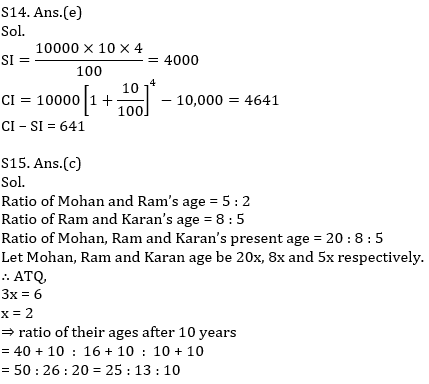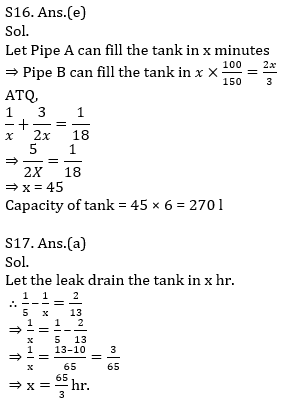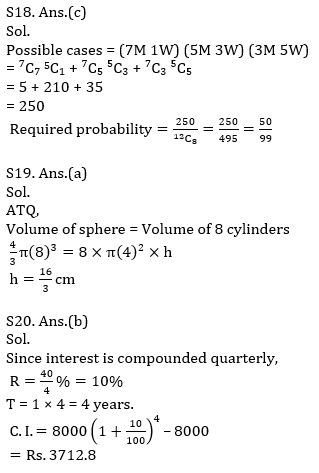#### Congratulations!Union Budget 2023-24: Free PDF